Total marks: --
Total time: --
INSTRUCTIONS
(1) Assume appropriate data and state your reasons
(2) Marks are given to the right of every question
(3) Draw neat diagrams wherever necessary

1 (a) With the help of neat block diagram, define open loop and closed loop control system.
4 M
1 (b) For a mechanical system shown in Fig. Q1(b) obtain force voltage analogous electrical network.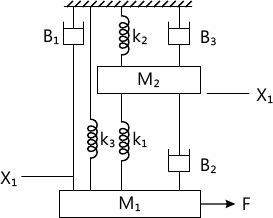8 M
1 (c) Draw the electrical network based on torque-current analogy and give all the performance equation for the Fig Q1(c).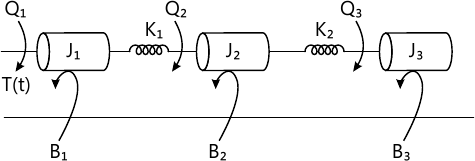8 M

2 (a) Define the following terms related to signal flow graph with a neat schematic:
i) Forward path
ii) Feedback loop
iii) Self loop
iv) Source node.
6 M
2 (b) Obtain the transfer function for the block diagram, shown in Fig. Q2(b). Using:
i) Block diagram reducing technique
ii) Mason's gain formula.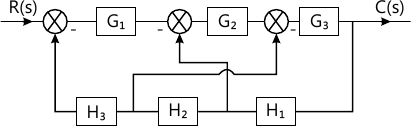8 M
2 (c) For the signal flow graph shown in Fig. Q2(c), find the overall transfer function by:
i) Block diagram reduction technique.
ii) Verify the result by Mason's gain formula.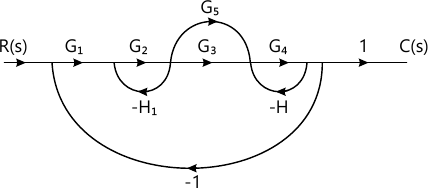8 M

3 (a) Define and derive the expression for: i) Rise time
ii) Peak overshoot of an under-damped second order control system subjected to step input.
6 M
3 (b) For a unit feedback control system with $G(s) = \dfrac {10 (s+2)} {s^2 (s+1)}$ Find: i) The static error coefficients ii) Steady state error when the input is $R(s)= \dfrac {3}{8} - \dfrac {2}{s^2} + \dfrac {1}{3s^3} .$
6 M
3 (c) A system is given by differential equation $\dfrac {d^2y}{dt^2} + 4\dfrac {dy}{dt} + 8y = 8x,$ where y=output and x=input. Determine: i) Peak overshoot ii) Settling time iii) Peak time for unit step input.
8 M

4 (a) Explain Routh-Hurwitz criterion for determining the stability of the system and mention its limitations.
6 M
4 (b) For a system s4+22s2+10s2+s+k=0, find kmar and ω at kmar.
6 M
4 (c) Determine the value of 'k' and 'b' so that the system whose open loop transfer function is: $G(s) = \dfrac {k(s+1)}{s^3+bs^2 + 3s+1}$ oscillates at a frequency of oscillations of 2 rad/sec.
8 M

5 (a) For a unity feedback system, the open loop transfer function is given by: $G(s) = \dfrac {K} {s(s+2)(s^2+6s+25)}$ i) Sketch the root locus for 0≤k≤∞ ii) At what value of 'k' the system becomes unstable
ii) At this point of instability, determine the frequency of oscillation of the system.
15 M
5 (b) Consider the system with $G(s)H(s) = \dfrac{k} {s(s+2)(s+4)}$ find whether s=-0.75 is point on root locus or not angle condition.
5 M

6 (a) Explain the procedure for investigating the stability using Nyquist criterion.
5 M
6 (b) For a certain control system: $G(s) H(s) = \dfrac {k} {s(s+2)(s+10)} .$ Sketch the Nyquist plot and hence calculate the range of value of 'k' for stability.
15 M

7 (a) Sketch the bode plot for the open loop transfer function: $G(s)H(s)= \dfrac {k(1+0.2s)(I+0.025s)}{s^3 (1+0.001s)(1+0.005s)} ,$ Find the range of 'k' for closed loop stability
14 M
7 (b) Explain the following as applied to bode plots:
i) Gain margin ii) Phase margin iii) Gain and phase cross over frequency.
6 M

8 (a) Define the following terms: i) State ii) State variable iii) State space iv) State transition.
4 M
8 (b) A system is described by the differential equation, $\dfrac {d^3y}{dt^3}+ \dfrac {3d^2y}{dt^2} + \dfrac {17dy}{dt}+ 5y = 10u(t)),$ where 'y' is the output and 'u' is input to the system. Determine the state space representation of the system.
6 M
8 (c) Obtain the state equations for the electrical network shown in Fig. Q8(c).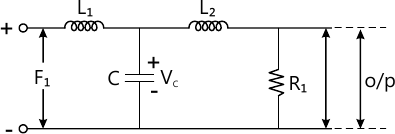10 M

More question papers from Control Systems